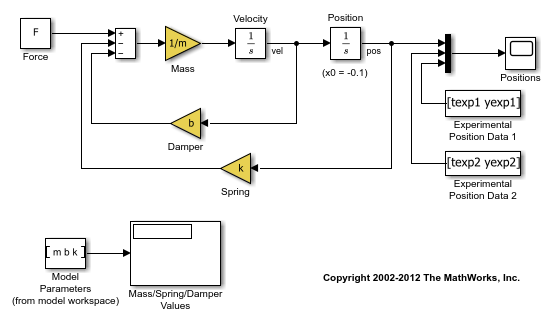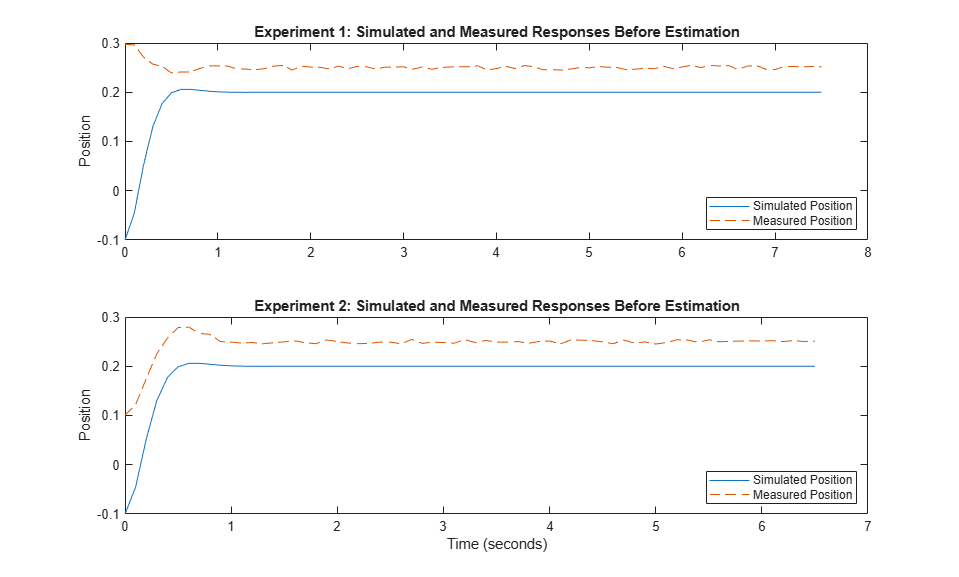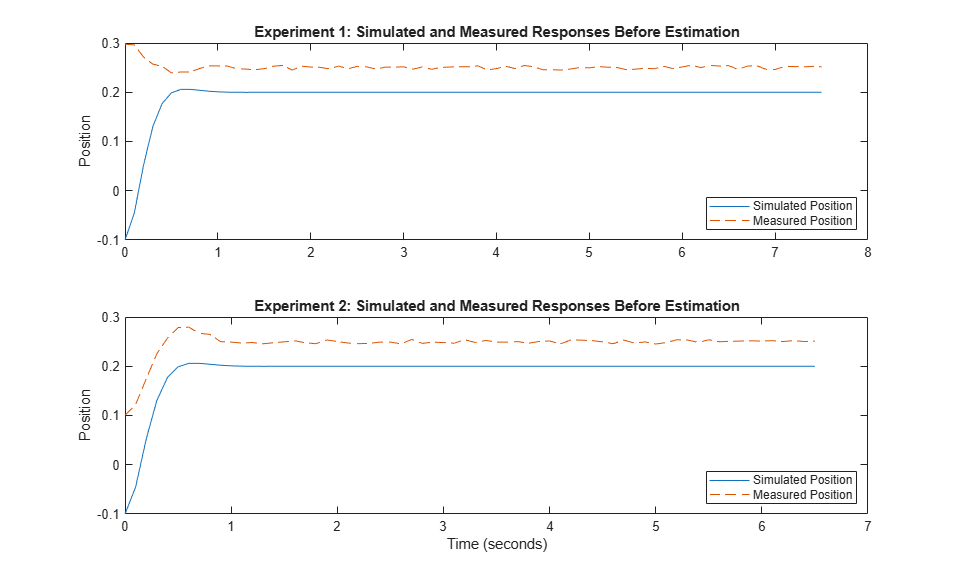Estimate Model Parameters Using Multiple Experiments (Code)

This example shows how to estimate model parameters from multiple sets of experimental data. You estimate the parameters of a mass-spring-damper system.

Open the Model and Get Experimental Data

This example uses the sdoMassSpringDamper model. The model includes two integrators to model the velocity and position of a mass in a mass-spring-damper system.

open_system('sdoMassSpringDamper');The variables texp1, yexp1, texp2, and yexp2 are loaded into the workspace. yexp1 and yexp2 describe the mass position for times texp1 and texp2, respectively.

Define the Estimation Experiments

Create a 2-element array of experiment objects to store the measured data for the two experiments.

Create an experiment object for the first experiment.

Exp = sdo.Experiment('sdoMassSpringDamper');

Create an object to store the measured mass position output.

MeasuredPos.Values    = timeseries(yexp1,texp1);
MeasuredPos.BlockPath = 'sdoMassSpringDamper/Position';
MeasuredPos.PortType  = 'outport';
MeasuredPos.PortIndex = 1;
MeasuredPos.Name      = 'Position';

Add the measured mass position data to the experiment as the expected output data.

Exp.OutputData = MeasuredPos;

Create an object to specify the initial state for the Velocity block. The initial velocity of the mass is 0 m/s.

sVel       = sdo.getStateFromModel('sdoMassSpringDamper','Velocity');
sVel.Value = 0;
sVel.Free  = false;

sVel.Free is set to false because the initial velocity is known and does not need to be estimated.

Create an object to specify the initial state for the Position block. Specify a guess for the initial mass position. Set the Free field of the initial position object to true so that it is estimated.

sPos       = sdo.getStateFromModel('sdoMassSpringDamper','Position');
sPos.Free  = true;
sPos.Value = -0.1;

Add the initial states to the experiment.

Exp.InitialStates = [sVel;sPos];

Create a two-element array of experiments. As the two experiments are identical except for the expected output data, copy the first experiment twice.

Exp = [Exp; Exp];

Modify the expected output data of the second experiment object in Exp.

Exp(2).OutputData.Values  = timeseries(yexp2,texp2);

Compare the Measured Output and the Initial Simulated Output

Create a simulation scenario using the first experiment and obtain the simulated output.

Simulator = createSimulator(Exp(1));
Simulator = sim(Simulator);

Search for the position signal in the logged simulation data.

SimLog   = find(Simulator.LoggedData,get_param('sdoMassSpringDamper','SignalLoggingName'));
Position = find(SimLog,'Position');

Obtain the simulated position signal for the second experiment.

Simulator   = createSimulator(Exp(2),Simulator);
Simulator   = sim(Simulator);
SimLog      = find(Simulator.LoggedData,get_param('sdoMassSpringDamper','SignalLoggingName'));
Position(2) = find(SimLog,'Position');

Plot the measured and simulated data.

The model response does not match the experimental output data.

subplot(211)
plot(...
Position(1).Values.Time,Position(1).Values.Data, ...
Exp(1).OutputData.Values.Time, Exp(1).OutputData.Values.Data,'--')
title('Experiment 1: Simulated and Measured Responses Before Estimation')
ylabel('Position')
legend('Simulated Position','Measured Position','Location','SouthEast')
subplot(212)
plot(...
Position(2).Values.Time,Position(2).Values.Data, ...
Exp(2).OutputData.Values.Time, Exp(2).OutputData.Values.Data,'--')
title('Experiment 2: Simulated and Measured Responses Before Estimation')
xlabel('Time (seconds)')
ylabel('Position')
legend('Simulated Position','Measured Position','Location','SouthEast')Specify Parameters to Estimate

Select the mass m, spring constant k, and damping coefficient b parameters from the model. Specify that the estimated values for these parameters must be positive.

p = sdo.getParameterFromModel('sdoMassSpringDamper', {'b', 'k', 'm'});
p(1).Minimum = 0;
p(2).Minimum = 0;
p(3).Minimum = 0;

Get the position initial state values to be estimated from the experiment.

s = getValuesToEstimate(Exp);

s contains two initial state objects, both for the Position block. Each object corresponds to an experiment in Exp.

Group the model parameters and initial states to be estimated together.

v = [p;s]

v(1,1) =

Name: 'b'
Value: 100
Minimum: 0
Maximum: Inf
Free: 1
Scale: 128
Info: [1x1 struct]

v(2,1) =

Name: 'k'
Value: 500
Minimum: 0
Maximum: Inf
Free: 1
Scale: 512
Info: [1x1 struct]

v(3,1) =

Name: 'm'
Value: 8
Minimum: 0
Maximum: Inf
Free: 1
Scale: 8
Info: [1x1 struct]

v(4,1) =

Name: 'sdoMassSpringDamper/Position'
Value: -0.1000
Minimum: -Inf
Maximum: Inf
Free: 1
Scale: 0.1250
dxValue: 0
dxFree: 1
Info: [1x1 struct]

v(5,1) =

Name: 'sdoMassSpringDamper/Position'
Value: -0.1000
Minimum: -Inf
Maximum: Inf
Free: 1
Scale: 0.1250
dxValue: 0
dxFree: 1
Info: [1x1 struct]

5x1 param.Continuous

Define the Estimation Objective

Create an estimation objective function to evaluate how closely the simulation output, generated using the estimated parameter values, matches the measured data.

Use an anonymous function with one input argument that calls the sdoMassSpringDamper_Objective function. P ass the anonymous function to sdo.optimize, which evaluates the function at each optimization iteration.

estFcn = @(v) sdoMassSpringDamper_Objective(v,Simulator,Exp);

The sdoMassSpringDamper_Objective function:

• Has one input argument that specifies the mass, spring constant and damper values as well as the initial mass position.

• Has one input argument that specifies the experiment object containing the measured data.

• Returns a vector of errors between simulated and experimental outputs.

The sdoMassSpringDamper_Objective function requires two inputs, but sdo.optimize requires a function with one input argument. To work around this, estFcn is an anonymous function with one input argument, v, but it calls sdoMassSpringDamper_Objective using two input arguments, v and Exp.

The sdo.optimize command minimizes the return argument of the anonymous function estFcn, that is, the residual errors returned by sdoMassSpringDamper_Objective. For more details on how to write an objective/constraint function to use with the sdo.optimize command, type help sdoExampleCostFunction at the MATLAB® command prompt.

To examine the estimation objective function in more detail, type edit sdoMassSpringDamper_Objective at the MATLAB command prompt.

type sdoMassSpringDamper_Objective
function vals = sdoMassSpringDamper_Objective(v,Simulator,Exp)
%SDOMASSSPRINGDAMPER_OBJECTIVE
%
%    The sdoMassSpringDamper_Objective function is used to compare model
%    outputs against experimental data.
%
%    vals = sdoMassSpringDamper_Objective(v,Exp)
%
%    The |v| input argument is a vector of estimated model parameter values
%    and initial states.
%
%    The |Simulator| input argument is a simulation object used
%    simulate the model with the estimated parameter values.
%
%    The |Exp| input argument contains the estimation experiment data.
%
%    The |vals| return argument contains information about how well the
%    model simulation results match the experimental data and is used by
%    the |sdo.optimize| function to estimate the model parameters.
%
%

% Copyright 2012-2015 The MathWorks, Inc.

%%
% Define a signal tracking requirement to compute how well the model output
% matches the experiment data. Configure the tracking requirement so that
% it returns the tracking error residuals (rather than the
% sum-squared-error) and does not normalize the errors.
%
r = sdo.requirements.SignalTracking;
r.Type      = '==';
r.Method    = 'Residuals';
r.Normalize = 'off';

%%
% Update the experiments with the estimated parameter values.
%
Exp  = setEstimatedValues(Exp,v);

%%
% Simulate the model and compare model outputs with measured experiment
% data.
%
Error = [];
for ct=1:numel(Exp)

Simulator = createSimulator(Exp(ct),Simulator);
Simulator = sim(Simulator);

SimLog  = find(Simulator.LoggedData,get_param('sdoMassSpringDamper','SignalLoggingName'));
Position = find(SimLog,'Position');

PositionError = evalRequirement(r,Position.Values,Exp(ct).OutputData.Values);

Error = [Error; PositionError(:)];
end

%%
% Return the residual errors to the optimization solver.
%
vals.F = Error(:);
end

Estimate the Parameters

Use the sdo.optimize function to estimate the actuator parameter values and initial state.

Specify the optimization options. The estimation function sdoMassSpringDamper_Objective returns the error residuals between simulated and experimental data and does not include any constraints, making this problem ideal for the lsqnonlin solver.

opt = sdo.OptimizeOptions;
opt.Method = 'lsqnonlin';

Estimate the parameters. Notice that the initial mass position is estimated twice, once for each experiment.

vOpt = sdo.optimize(estFcn,v,opt)
Optimization started 01-Sep-2021 16:56:34

First-order
Iter F-count        f(x)      Step-size  optimality
0     11     0.777696            1
1     22   0.00413099        3.696      0.00648
2     33   0.00118327       0.3194      0.00243
3     44    0.0011106      0.06718     5.09e-05
Local minimum found.

Optimization completed because the size of the gradient is less than
the value of the optimality tolerance.

vOpt(1,1) =

Name: 'b'
Value: 58.1959
Minimum: 0
Maximum: Inf
Free: 1
Scale: 128
Info: [1x1 struct]

vOpt(2,1) =

Name: 'k'
Value: 399.9452
Minimum: 0
Maximum: Inf
Free: 1
Scale: 512
Info: [1x1 struct]

vOpt(3,1) =

Name: 'm'
Value: 9.7225
Minimum: 0
Maximum: Inf
Free: 1
Scale: 8
Info: [1x1 struct]

vOpt(4,1) =

Name: 'sdoMassSpringDamper/Position'
Value: 0.2995
Minimum: -Inf
Maximum: Inf
Free: 1
Scale: 0.1250
dxValue: 0
dxFree: 1
Info: [1x1 struct]

vOpt(5,1) =

Name: 'sdoMassSpringDamper/Position'
Value: 0.0994
Minimum: -Inf
Maximum: Inf
Free: 1
Scale: 0.1250
dxValue: 0
dxFree: 1
Info: [1x1 struct]

5x1 param.Continuous

Compare the Measured Output and the Final Simulated Output

Update the experiments with the estimated parameter values.

Exp  = setEstimatedValues(Exp,vOpt);

Obtain the simulated output for the first experiment.

Simulator   = createSimulator(Exp(1),Simulator);
Simulator   = sim(Simulator);
SimLog      = find(Simulator.LoggedData,get_param('sdoMassSpringDamper','SignalLoggingName'));
Position(1) = find(SimLog,'Position');

Obtain the simulated output for the second experiment.

Simulator   = createSimulator(Exp(2),Simulator);
Simulator   = sim(Simulator);
SimLog      = find(Simulator.LoggedData,get_param('sdoMassSpringDamper','SignalLoggingName'));
Position(2) = find(SimLog,'Position');

Plot the measured and simulated data.

The model response using the estimated parameter values matches the output data for the experiments.

subplot(211)
plot(...
Position(1).Values.Time,Position(1).Values.Data, ...
Exp(1).OutputData.Values.Time, Exp(1).OutputData.Values.Data,'--')
title('Experiment 1: Simulated and Measured Responses After Estimation')
ylabel('Position')
legend('Simulated Position','Measured Position','Location','NorthEast')
subplot(212)
plot(...
Position(2).Values.Time,Position(2).Values.Data, ...
Exp(2).OutputData.Values.Time, Exp(2).OutputData.Values.Data,'--')
title('Experiment 2: Simulated and Measured Responses After Estimation')
xlabel('Time (seconds)')
ylabel('Position')
legend('Simulated Position','Measured Position','Location','SouthEast')Update the Model Parameter Values

Update the model m, k, and b parameter values. Do not update the model initial position value as this is dependent on the experiment.

sdo.setValueInModel('sdoMassSpringDamper',vOpt(1:3));

Close the model

bdclose('sdoMassSpringDamper')